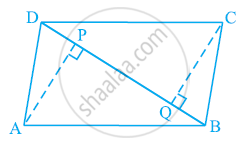# ABCD is a parallelogram and AP and CQ are perpendiculars from vertices A and C on diagonal BD (See the given figure). Show that (i) ΔAPB ≅ ΔCQD (ii) AP = CQ - Mathematics

ABCD is a parallelogram and AP and CQ are perpendiculars from vertices A and C on diagonal BD (See the given figure). Show that

(i) ΔAPB ≅ ΔCQD

(ii) AP = CQ#### Solution

(i) In ΔAPB and ΔCQD,

∠APB = ∠CQD (Each 90°)

AB = CD (Opposite sides of parallelogram ABCD)

∠ABP = ∠CDQ (Alternate interior angles for AB || CD)

∴ ΔAPB ≅ ΔCQD (By AAS congruency)

(ii) By using the above result

ΔAPB ≅ ΔCQD, we obtain

AP = CQ (By CPCT)

Concept: Another Condition for a Quadrilateral to Be a Parallelogram
Is there an error in this question or solution?
Chapter 8: Quadrilaterals - Exercise 8.1 [Page 147]

#### APPEARS IN

NCERT Class 9 Maths